## Introduction

The aim of this tutorial is to solve the EEG forward problem using two different numerical methods, namely the Boundary Element Method (BEM) and the Finite Element Method (FEM).

## Background

The EEG/MEG signals measured on the scalp do not directly reflect the location of the activated neurons. To reconstruct the location and the time-course or spectral content of a source in the brain, various source-localization methods are available. You can read more about the different methods in review papers suggested here.

The level of the activity at a source location is estimated from

1. the EEG/MEG activity measured on (around) the scalp
2. the spatial arrangement of the electrodes/sensors (channel positions),
3. the geometrical and electrical/magnetic properties of the head (head model)
4. the location of the source (source model)

Using this information, source estimation comprises two major steps: (1) Estimation of the potential or field distribution for a known source and for a known model of the head is referred to as forward modeling. (2) Estimation of the unknown sources corresponding to the measured EEG or MEG is referred to as inverse modeling.

The forward solution can be computed when the head model, the channel positions and the source is given. For distributed source models and for scanning approaches (such as beamforming), the source model is discretizing the brain volume into a volumetric or surface grid. When the forward solution is computed, the lead field matrix (= channels X source points matrix) is calculated for each grid point taking into account the head model and the channel positions.

A prerequisite of forward modeling is that the geometrical description of all elements (channel positions, head model and source model) is registered in the same coordination system with the same units. There are different “conventions” how to define a coordinate system, but the precise coordinate system is not relevant, as long as all data is expressed in it consistently (i.e. in the same coordinate system). Here read more about how the different head and mri coordinate systems are defined. The MEG sensors by default are defined relative to anatomical landmarks of the head (the fiducial coils), therefore when the anatomical images are also aligned to these landmarks, the MEG sensors do not need to be re-aligned. EEG data is typically not aligned to the head, therefore, the electrodes have to be re-aligned prior to source-reconstruction (see also this faq and this example).Figure 1. Major steps in source reconstruction

## Procedure

As already mentioned, the goal of this session is to solve the EEG forward problem, more precisely we want to compute EEG leadfields so that the inverse problem can be solved in the next session (inverse problem). In order to compute leadfields, there are 9 main steps that have to be followed.

1. Load and read the anatomical data, namely a T1-MRI (ft_read_mri);
2. Align the MRI to the electrodes. As the electrodes are expressed in the CTF coordinate system, we translate the MRI in the CTF coordinate system (ft_volumerealign);
3. Reslice the MRI image so that the voxels of the anatonical data are homogeneous (i.e. the size of the voxel is the same into each direction). This step will facilitate the segmentation step. (ft_volumereslice)
4. Segment the MRI: 3 compartments for BEM (scalp, skull, brain) and 5 compartments for FEM (scalp, skull, CSF, grey matter and white matter) (ft_volumesegment);
5. then we create the mesh: triangulated surface mesh for BEM and hexahedral volume mesh for FEM (ft_prepare_mesh).
6. Create the headmodels (headmodel_bem and headmodel_fem) where geometrical and electrical information are merged together (ft_prepare_headmodel);
7. Align the electrodes to the MRI (ft_electroderealign);
8. The sourcemodel is created, where the location of the sources is restrained to the brain compartment (from the BEM mesh) (ft_prepare_sourcemodel);
9. Leadfields can be computed (ft_prepare_leadfield).

The first 3 steps are the same for BEM and FEM. Steps from 4 to 8 differ between BEM and FEM. A more detailed description of these steps is following.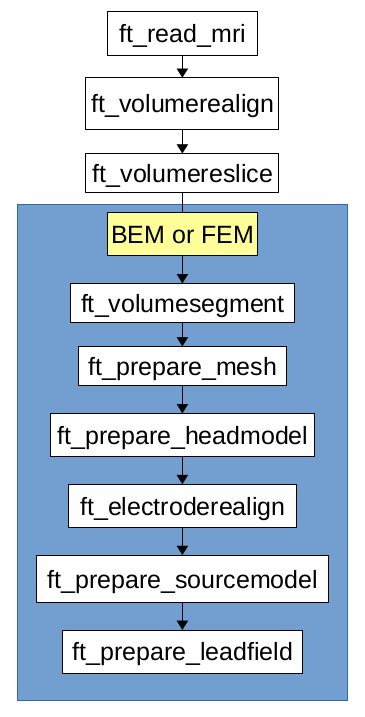Figure1: pipeline for forward computation, in the blue box there are the steps which differ between BEM and FEM

## 1. Read the MRI

``````mri_orig = ft_read_mri('subject01.nii');
``````

Visualize the MRI

``````cfg=[];
ft_sourceplot(cfg,mri_orig);
``````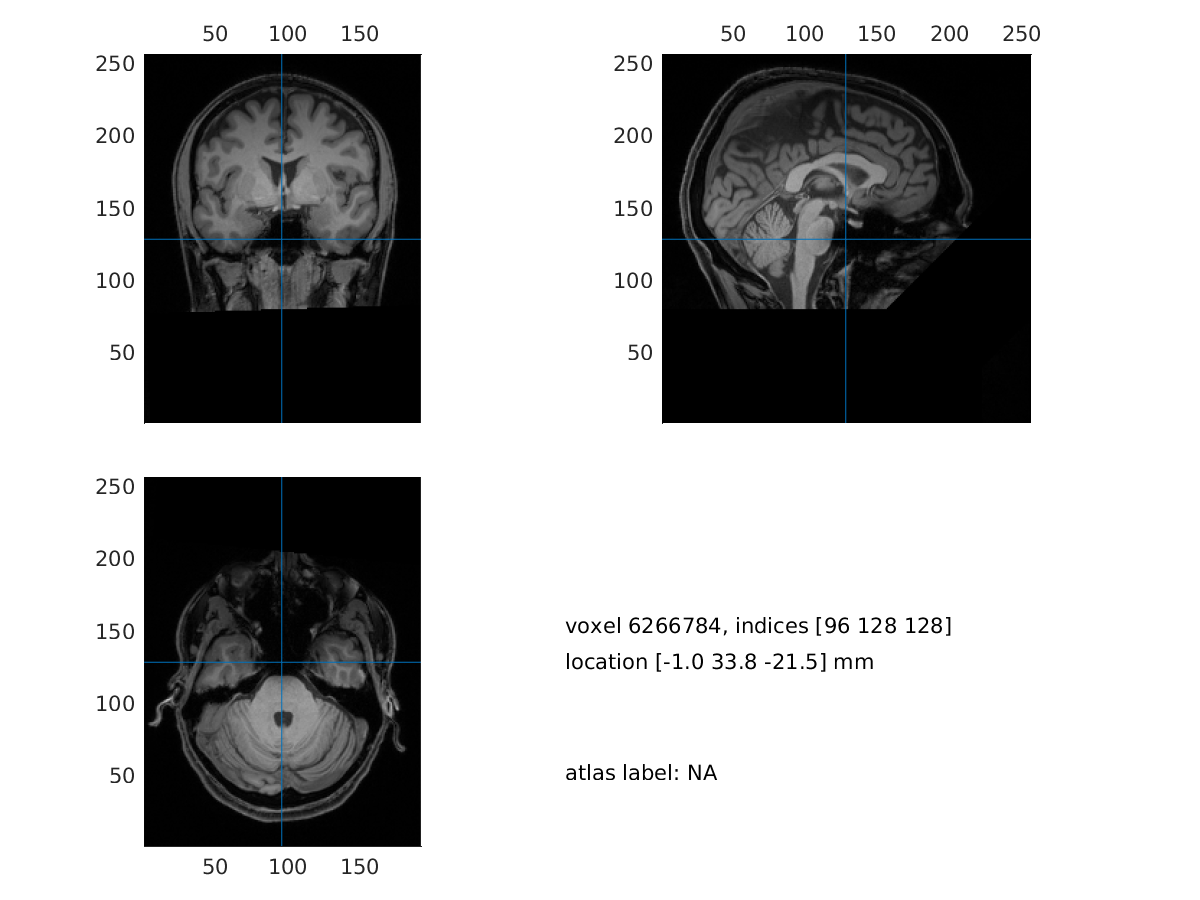Figure2: visualization of the MRI

## 2. Realign the MRI

In this step we will interactively align the MRI to the CTF space. We will be asked to identify the three CTF landmarks (nasion, NAS; right pre-auricular point, RPA; left pre-auricular point, LPA) in the MRI.

``````cfg = [];
cfg.method = 'interactive';
cfg.coordsys = 'ctf';
mri_realigned = ft_volumerealign(cfg, mri_orig);
``````

We can visualize the realigned MRI

``````cfg = [];
ft_sourceplot(cfg, mri_realigned);
``````Figure3: visualization of the realigned MRI

## 3. Reslice the MRI

``````cfg = [];
mri_resliced = ft_volumereslice(cfg, mri_realigned);
``````

We can visualize the resliced MRI

``````cfg = [];
ft_sourceplot(cfg, mri_resliced);
``````

## 4A. Segment the MRI

``````cfg = [];
cfg.output = {'brain','skull', 'scalp'};
mri_segmented_3_compartment = ft_volumesegment(cfg, mri_resliced);
``````

Visualize the segmentation

``````seg_i = ft_datatype_segmentation(mri_segmented_3_compartment,'segmentationstyle','indexed');

cfg = [];
cfg.funparameter = 'seg';
cfg.funcolormap  = gray(4); % distinct color per tissue
cfg.location     = 'center';
cfg.atlas        = seg_i;
ft_sourceplot(cfg, seg_i);
``````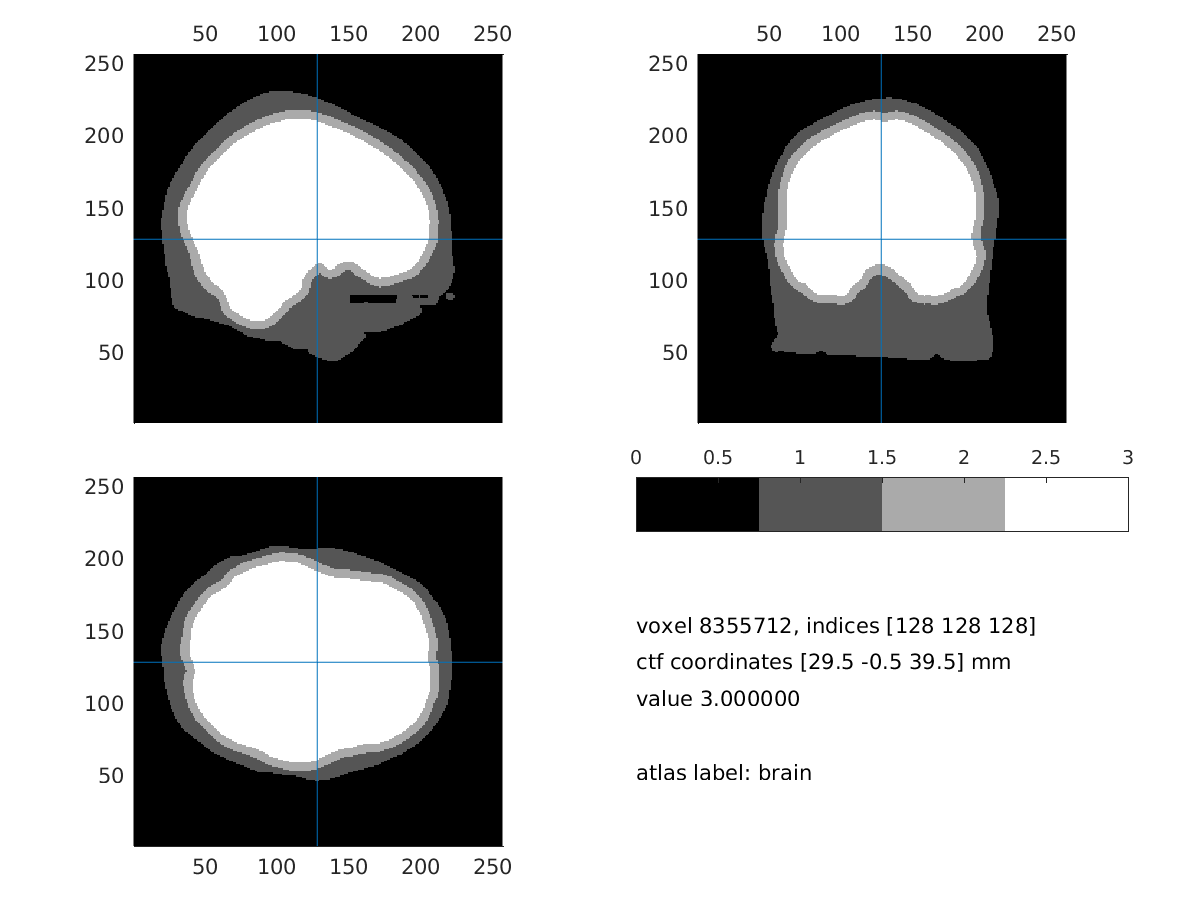Figure4: 3 compartment segmentation output

## 5A. Create the mesh

``````cfg = [];
cfg.tissue = {'brain','skull','scalp'};
cfg.numvertices = [3000 2000 1000];
mesh_bem=ft_prepare_mesh(cfg,mri_segmented_3_compartment);
``````

Visualize the mesh and the electrode

``````load elec; %load the electrodes
figure, ft_plot_mesh(mesh_bem(1),'surfaceonly','yes','vertexcolor','none','facecolor',...
'skin','facealpha',0.5,'edgealpha',0.1)
ft_plot_mesh(mesh_bem(2),'surfaceonly','yes','vertexcolor','none','facecolor',...
'skin','facealpha',0.5,'edgealpha',0.1)
ft_plot_mesh(mesh_bem(3),'surfaceonly','yes','vertexcolor','none','facecolor',...
'skin','facealpha',0.5,'edgealpha',0.1)
hold on, ft_plot_sens(elec, 'style', '*g');
``````Figure5: 3 compartment mesh with electrodes

## 6A. Create the headmodel

``````cfg = [];
cfg.method ='dipoli'; % You can also specify 'bemcp', or another method.
headmodel_bem       = ft_prepare_headmodel(cfg, mesh_bem);
``````

In Windows the method ‘dipoli’ does not work. You can either load “headmodel_bem” and continue with this tutorial, or explore other BEM method like ‘bemcp’. If you use ‘bemcp’, the conductivity field has a different order: {‘brain’, ‘skull’, ‘skin’}.

## 7A. Align the electrodes

If the electrodes are not well aligned with the mesh, we can realign them with:

``````cfg = [];
cfg.method    = 'interactive';
cfg.elec      = elec;
cfg.headshape = headmodel_bem.bnd;
elec = ft_electroderealign(cfg);
``````

Check the alignment visually.

``````figure;
ft_plot_axes(mesh_bem)
hold on;
ft_plot_mesh(mesh_bem.bnd(1),'surfaceonly','yes','vertexcolor','none','facecolor',...
'skin','facealpha',0.5,'edgealpha',0.1)
ft_plot_sens(elec,'style', '.k');
``````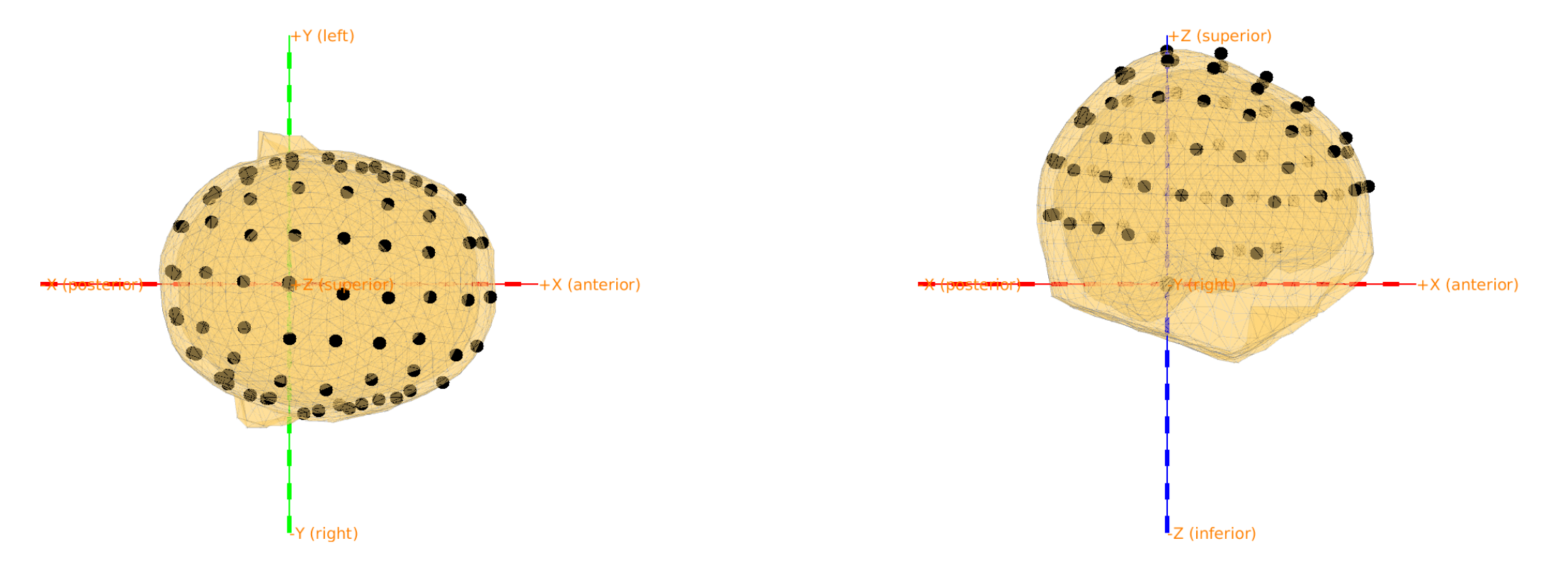Figure6: mesh, electrodes and axes.

## 8A. Create the sourcemodel

``````cfg = [];
cfg.resolution = 7.5;
cfg.threshold = 0.1;
cfg.smooth = 5;
cfg.headmodel = headmodel_bem;
cfg.inwardshift = 1; %shifts dipoles away from surfaces
sourcemodel = ft_prepare_sourcemodel(cfg);
``````

Visualize the sourcemodel

``````figure, ft_plot_mesh(sourcemodel.pos(sourcemodel.inside,:))
hold on, ft_plot_mesh(mesh_bem.bnd(1),'surfaceonly','yes','vertexcolor','none','facecolor',...
'skin','facealpha',0.5,'edgealpha',0.1)
``````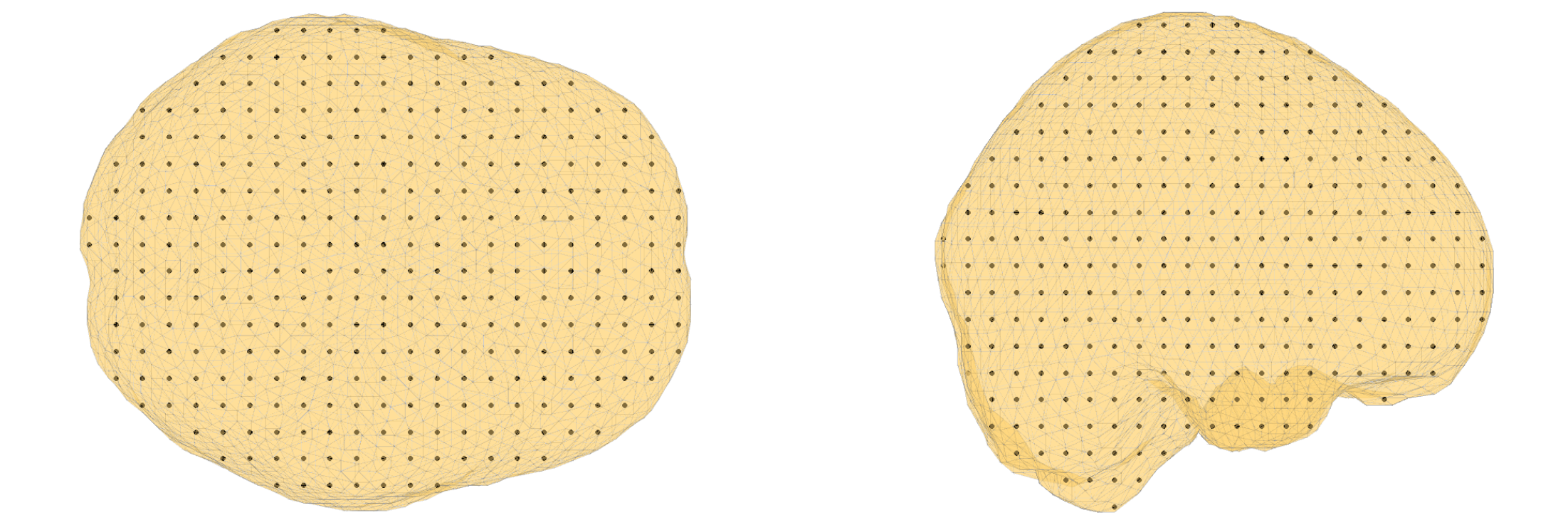Figure7: sourcemodel on the brain compartment

Save the sourcemode

``````save sourcemodel sourcemodel;
``````

## 9A. Compute the leadfield

``````cfg = [];
cfg.grid = sourcemodel;
cfg.headmodel= headmodel_bem;
cfg.elec = elec;
cfg.reducerank = 3;
leadfield_bem = ft_prepare_leadfield(cfg);
``````

## 4B. Segment the MRI

``````cfg           = [];
cfg.output         = {'scalp','skull','csf','gray','white'};
cfg.brainsmooth    = 1;
cfg.scalpthreshold = 0.11;
cfg.skullthreshold = 0.15;
cfg.brainthreshold = 0.15;
mri_segmented_5_compartment = ft_volumesegment(cfg, mri_resliced);
``````

Visualize the segmentation result

``````seg_i = ft_datatype_segmentation(mri_segmented_5_compartment,'segmentationstyle','indexed');

cfg              = [];
cfg.funparameter = 'seg';
cfg.funcolormap  = gray(5); % distinct color per tissue
cfg.location     = 'center';
cfg.atlas        = seg_i;    % the segmentation can also be used as atlas
ft_sourceplot(cfg, seg_i);
``````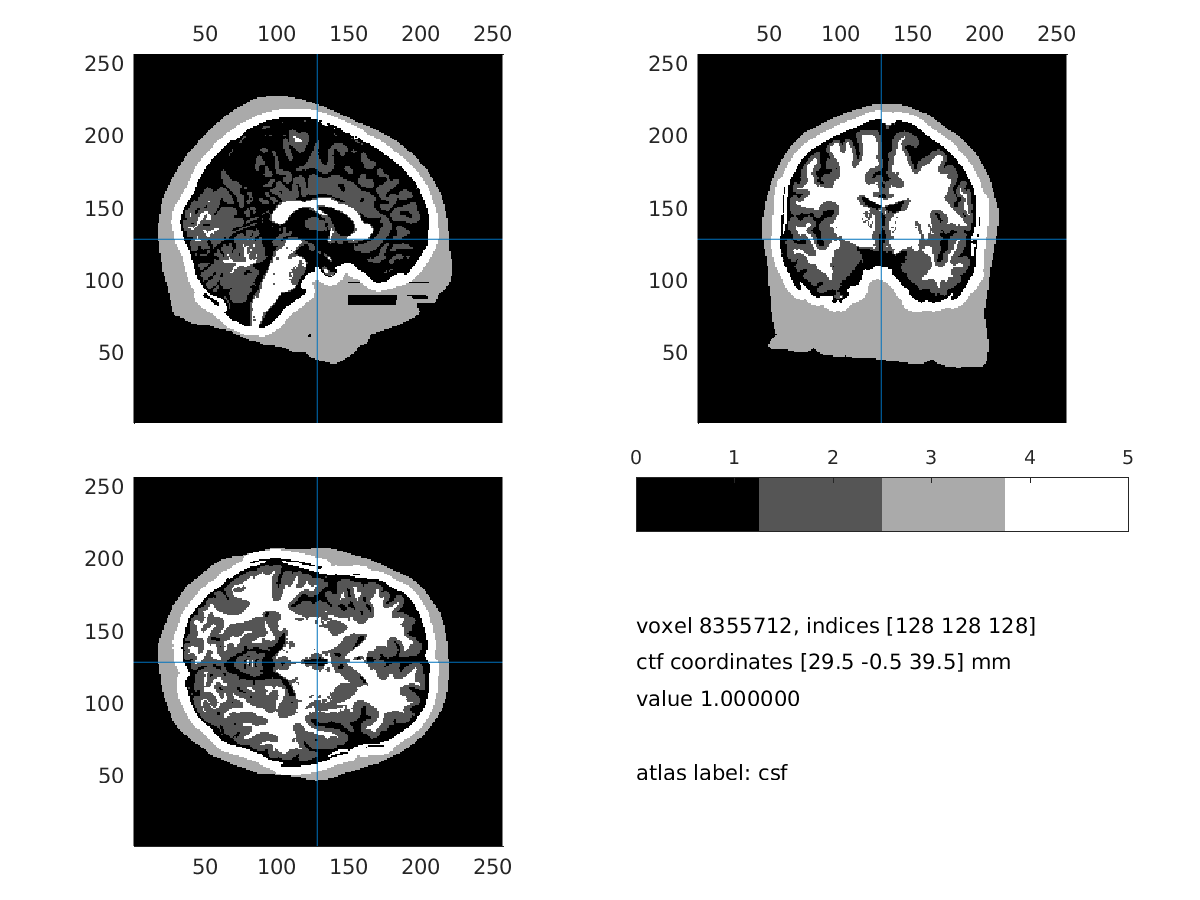_Figure8: 5 compartment segmentation output _

## 5B. Create the mesh

``````cfg        = [];
cfg.shift  = 0.3;
cfg.method = 'hexahedral';
cfg.resolution = 1; % this is in mm
mesh_fem = ft_prepare_mesh(cfg,mri_segmented_5_compartment);
``````

## 6B. Create the headmodel

``````cfg = [];
cfg.method = 'simbio';
cfg.conductivity = [0.43 0.0024 1.79 0.14 0.33]; % same as tissuelabel in vol_simbio
cfg.tissuelabel = {'scalp', 'skull', 'csf', 'gray','white'};
headmodel_fem = ft_prepare_headmodel(cfg, mesh_fem);
``````

Visualize the headmodel and the electrodes (it might take time and memory)

``````% csf: 1, gm: 2, scalp: 3, skull: 4, wm: 5
ts = 3;
figure
mesh2 =[];
mesh2.hex = headmodel_fem.hex(headmodel_fem.tissue==ts,:); %mesh2.hex(1:size(mesh2.hex),:);
mesh2.pos = headmodel_fem.pos;
mesh2.tissue = headmodel_fem.tissue(headmodel_fem.tissue==ts,:); %mesh.tissue(1:size(mesh2.hex),:);

mesh_ed = mesh2edge(mesh2);
patch('Faces',mesh_ed.poly,...
'Vertices',mesh_ed.pos,...
'FaceAlpha',.5,...
'LineStyle','none',...
'FaceColor',[1 1 1],...
'FaceLighting','gouraud');

xlabel('coronal');
ylabel('sagital');
zlabel('axial')
camlight;
axis on;

ft_plot_sens(elec, 'style', '*g');
``````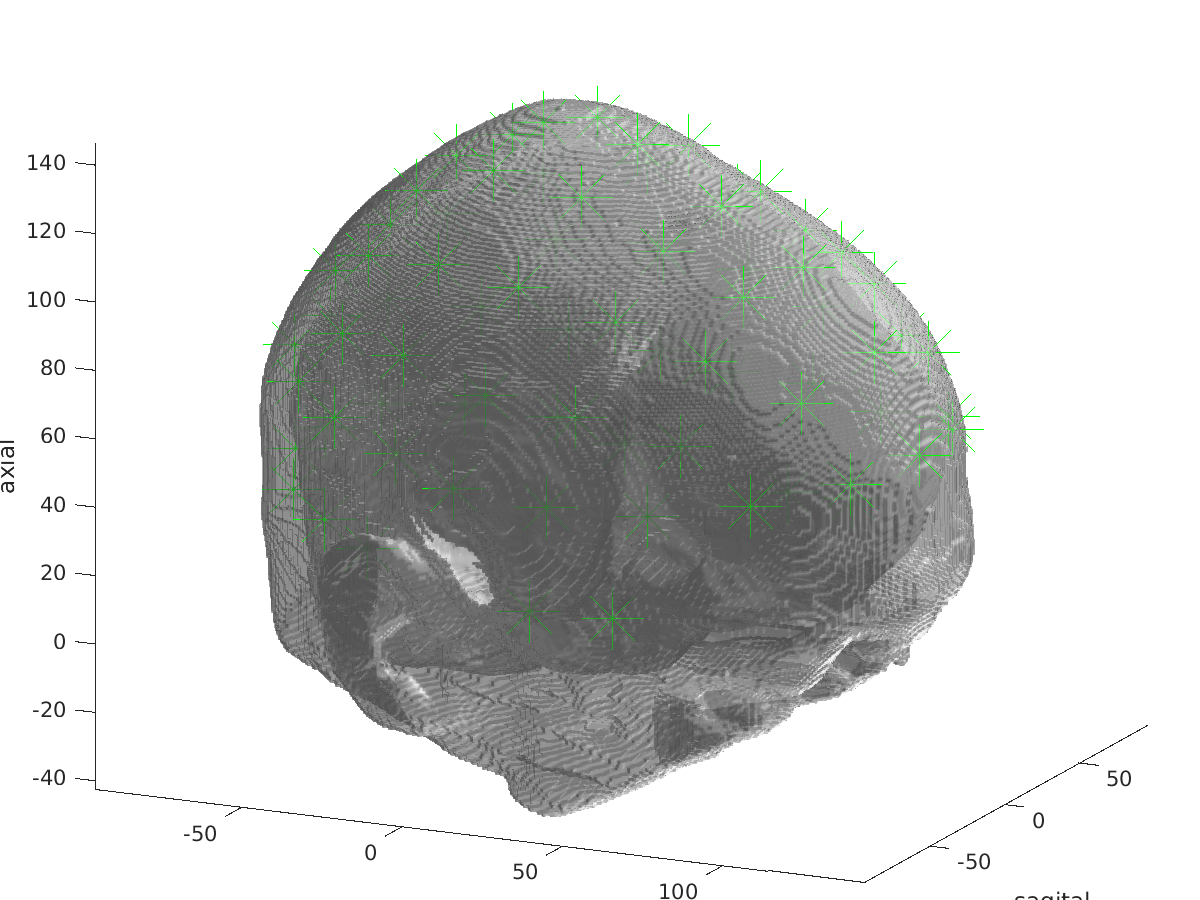Figure9: visualization of headmodel_fem and electrodes

## 7B. Align the electrodes

If the electrodes are not well aligned with the mesh, we can realign them with:

``````cfg           = [];
cfg.method    = 'interactive';
cfg.elec      = elec;
cfg.headshape = headmodel_fem;
elec = ft_electroderealign(cfg);
``````

## 8B. Create the sourcemodel

We will use the sourcemodel already generated in 7A.

``````load('sourcemodel.mat');
``````

## 9B. Compute the leadfield

Please DO NOT run ft_prepare_vol_sens in this tutorial session! It will take too much time and memory. Load “headmodel_fem_tr”.

``````%% compute the transfer matrix
[headmodel_fem_tr, elec] = ft_prepare_vol_sens(headmodel_fem, elec);

%% compute the leadfield
cfg = [];
cfg.grid = sourcemodel;
cfg.headmodel= headmodel_fem_tr;
cfg.elec = elec;
cfg.reducerank = 3;
leadfield_fem = ft_prepare_leadfield(cfg);
``````

## Summary and Comments

This tutorial was about the computation of leadfields that could be feed into the inverse problem which will be explain i Inverse problem.

This tutorial was last tested on 27-08-2017 by Maria Carla Piastra on Ubuntu, Matlab 2015b.